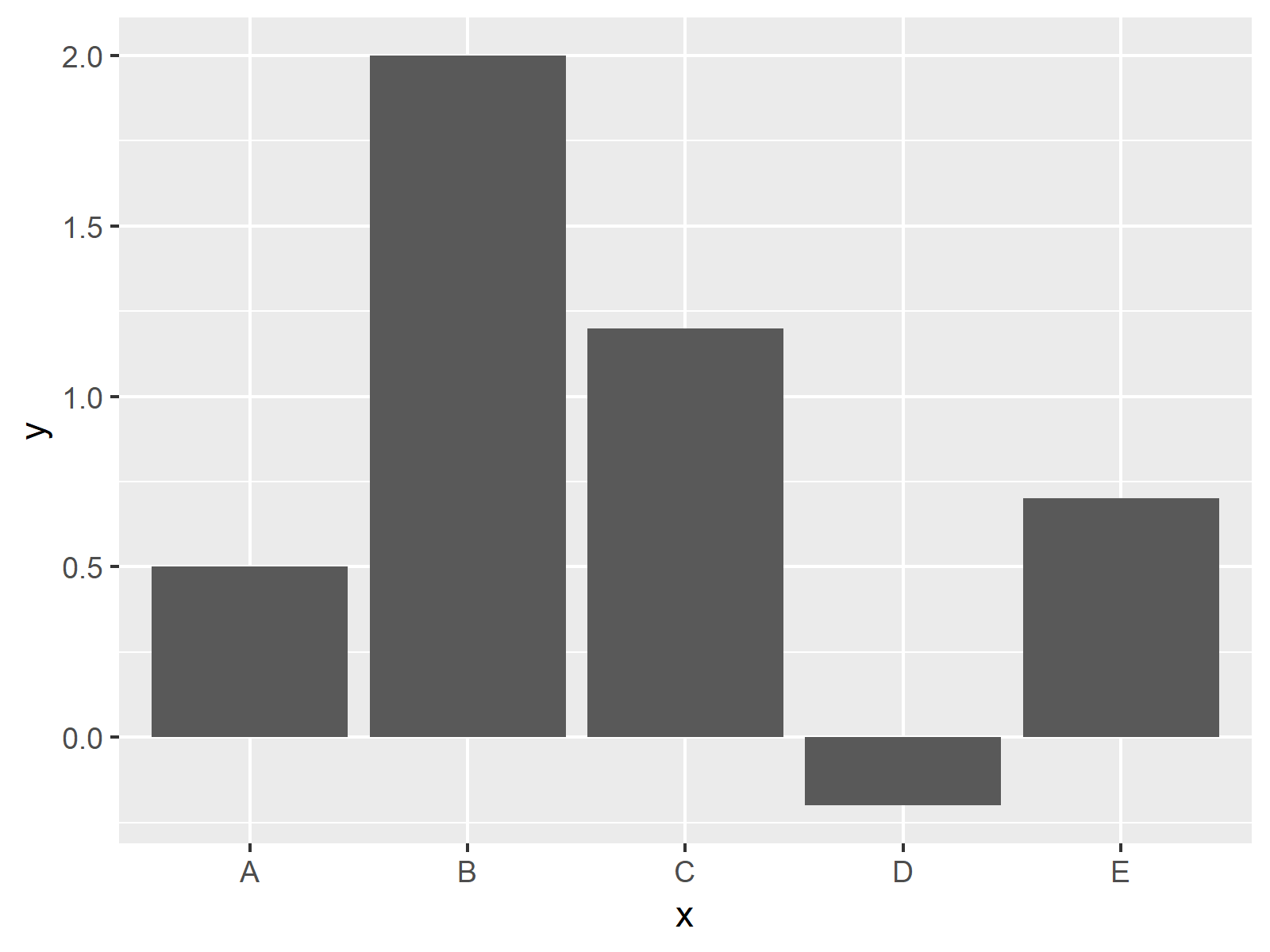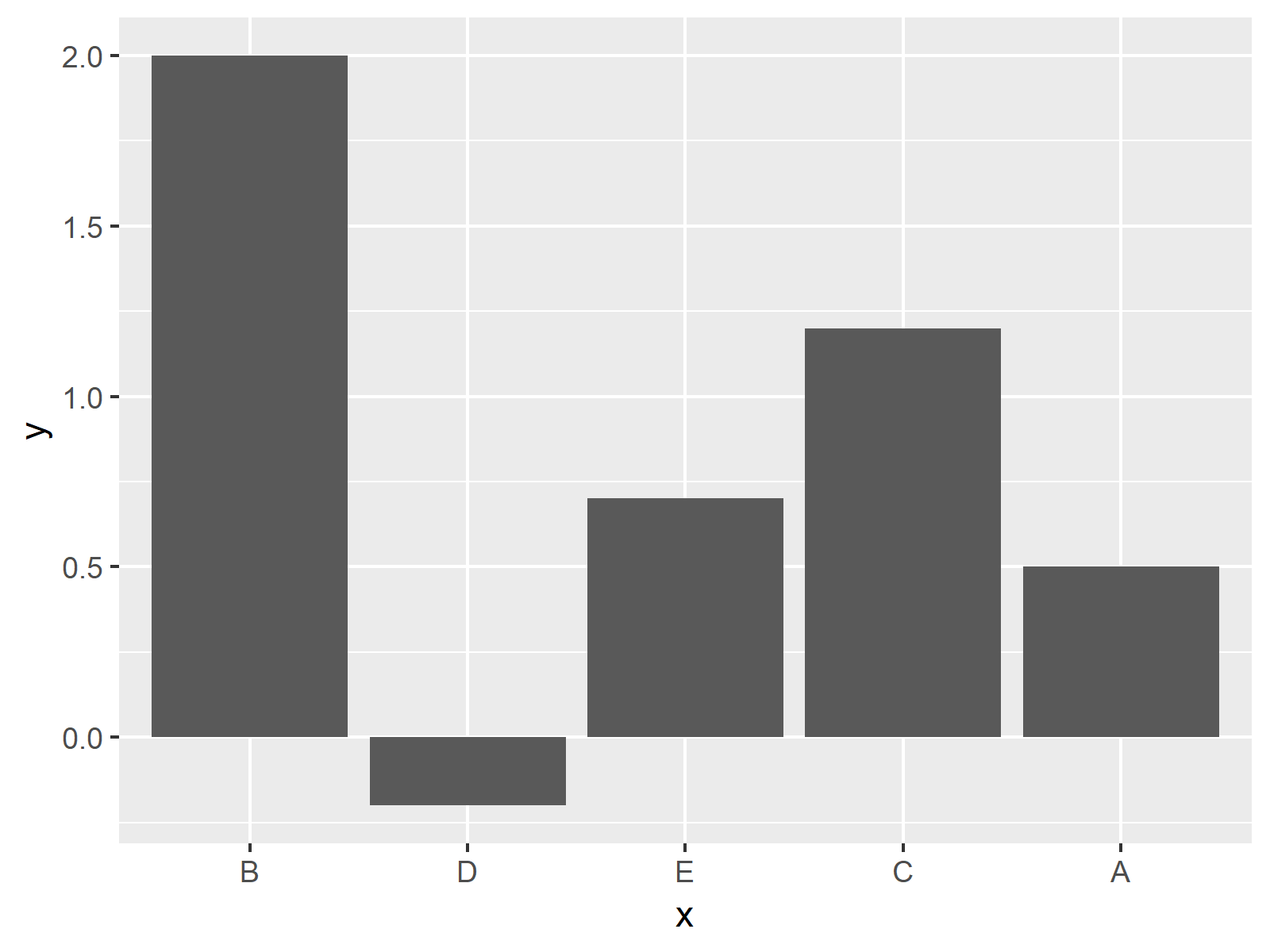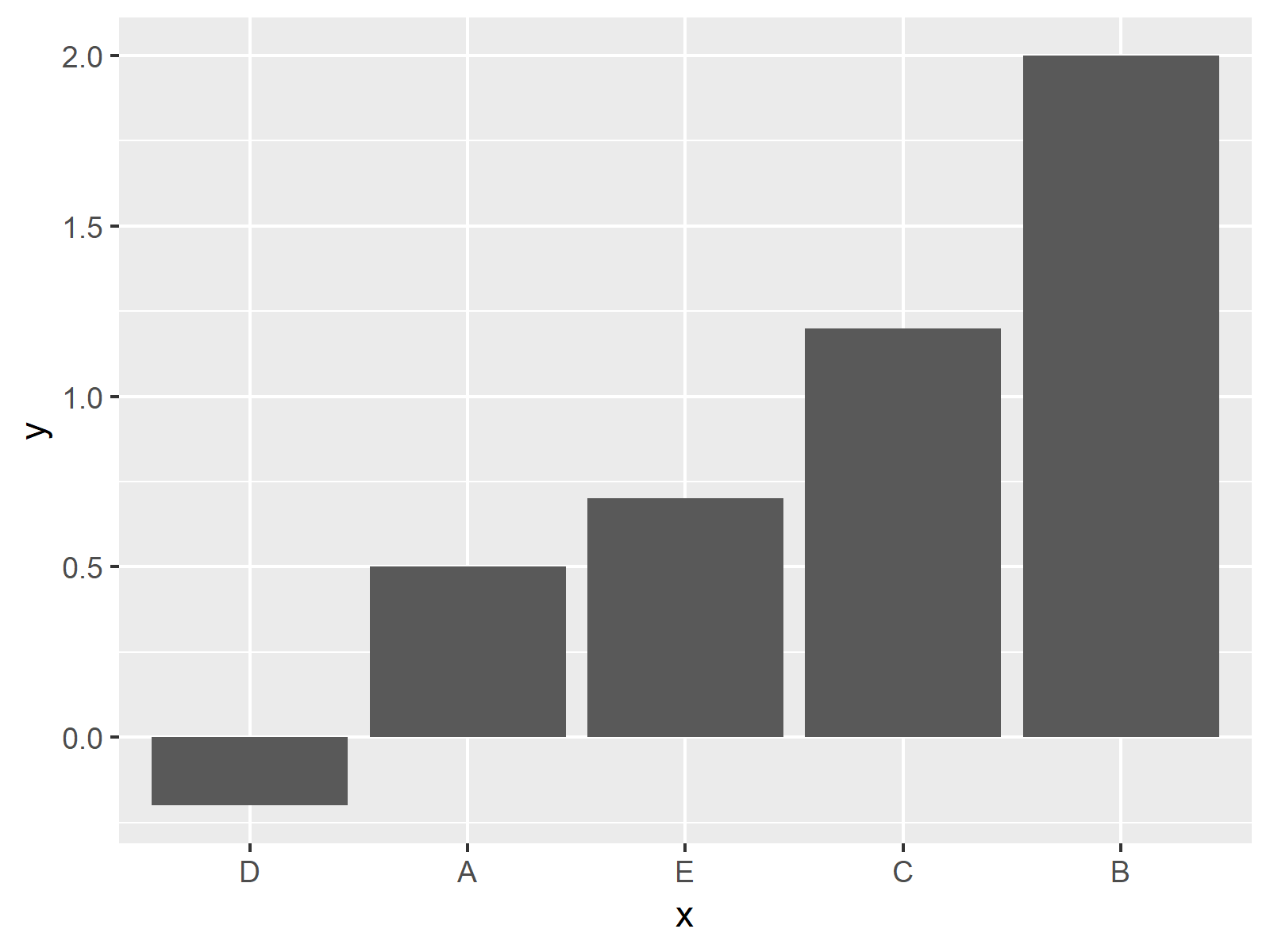# Order Bars of ggplot2 Barchart in R (4 Examples)

In this R tutorial you’ll learn how to order the bars of a ggplot2 barchart.

The content is structured as follows:

Here’s how to do it!

## Creation of Example Data

First, we need to create some example data to illustrate the following examples:

```data <- data.frame(x = c("A", "B", "C", "D", "E"),            # Create example data
y = c(0.5, 2, 1.2, -0.2, 0.7))```

Furthermore, we need to install and load the ggplot2 package to R:

```install.packages("ggplot2")                                   # Install ggplot2 package

Now, we can draw a basic barplot with the following R code:

```ggplot(data, aes(x, y)) +                                     # Create basic barchart
geom_bar(stat = "identity")```Figure 1: Basic Barchart in ggplot2 R Package.

Figure 1 shows the output of the previous R code – An unordered ggplot2 Barplot in R.

## Example 1: Ordering Bars Manually

If we want to change the order of the bars manually, we need to modify the factor levels of our ordering column. We can do that with the following R syntax:

```data1 <- data                                                 # Replicate original data
data1\$x <- factor(data1\$x,                                    # Change ordering manually
levels = c("B", "D", "E", "C", "A"))```

If we now use exactly the same ggplot2 syntax as before, we get the following plot:

```ggplot(data1, aes(x, y)) +                                    # Manually ordered barchart
geom_bar(stat = "identity")```Figure 2: Manual Order of Bars.

Figure 2 illustrates the new ordering of our barchart.

## Example 2: Barchart with Increasing Order

Let’s assume that we want to sort our barplot by the size of the bars. Then we can easily use the sort function to order the factor levels according to the values of our bars:

```data2 <- data                                                 # Replicate original data
data2\$x <- factor(data2\$x,                                    # Factor levels in increasing order```

levels = data2\$x[order(data2\$y)])

```ggplot(data2, aes(x, y)) +                                    # Increasingly ordered barchart
geom_bar(stat = "identity")```Figure 3: Increasing Order of Bars.

As you can see in Figure 3, our bars were sorted in decreasing order.

## Example 3: Barchart with Decreasing Order

Of course, you could also do the sorting the other way around in reversed order. The following R code sorts our bars in decreasing order:

```data3 <- data                                                 # Replicate original data
data3\$x <- factor(data3\$x,                                    # Factor levels in decreasing order
levels = data3\$x[order(data3\$y, decreasing = TRUE)])```
```ggplot(data3, aes(x, y)) +                                    # Decreasingly ordered barchart
geom_bar(stat = "identity")```Figure 4: Decreasing Order of Bars.

That’s it!

## Video, Further Resources & Summary

Would you like to learn more about the ordering of bargraphs using ggplot2? Then you might have a look at the following video of my YouTube channel. In the video, I illustrate the content of this post.

Please accept YouTube cookies to play this video. By accepting you will be accessing content from YouTube, a service provided by an external third party.If you accept this notice, your choice will be saved and the page will refresh.

Furthermore, I can recommend having a look at some of the related articles of this website. A selection of articles about the ordering of factors is listed here.

You learned in this article how to reorder factors to plot the bars of a ggplot in a specified axis order in R programming.

Note that it would be possible to use similar R codes to reorder or reverse the axis orders of other types of graphs showing discrete or categorical variables such as boxplots or heatmaps.

Subscribe to the Statistics Globe Newsletter

•Manjinder
December 30, 2020 12:08 am

Hi Joachim,

I am trying to make a legend for my data that would reflect bar colours. When I add “col = status” it adds the legend but with dark grey colour inside. Any help would be appreciated?

The code I am using is:
p1 <- ggplot(d1, aes(x= reorder(gene, -fold_change), y= fold_change, fill = status, color = status)) +
geom_bar(position = position_dodge(0.9), stat = "identity", width = 0.8) +
geom_errorbar( aes(ymin=fold_change-se, ymax=fold_change+se), width=0.2, position = position_dodge(0.8), colour="black", alpha=0.9, size=0.5) +
ggtitle("Gene expression") +
xlab(NULL) +
ylab("Fold change") +
theme_classic() +
theme(axis.text=element_text(size=12), axis.title=element_text(size=14,face="bold"))+
theme(axis.text = element_text(colour = "black", size = rel(1.15))) +
theme(panel.background = element_rect(fill = "white"), plot.margin = margin(0.5, 0.5, 0.5, 0.5, "cm"), plot.background = element_rect(fill = "grey90",colour = "black",size = 1)) +
theme(legend.title = element_text(color = "black",size = 15))+
theme(plot.title = element_text(size = 20, face = "bold")) +
guides(fill = FALSE)

p1
Thank you
Manjinder

•December 30, 2020 6:46 am

Hey Manjinder,

Thank you for the comment. I think the reason for this is the last line of your code (i.e. ‘+ guides(fill = FALSE)’). If you remove this line it should work.

I hope you’ll have a happy new year!

Joachim

•Manjinder
January 2, 2021 5:15 am

Hi Joachim,

Now my graph looks pretty except for one issue I am trying to fix. I have two bars orange and green, right next to each other for “Bleed” and “No bleed” samples. By default, bleed bars come first, and I want to change it to make the “No-bleed” bar appear first. Is there any way to do that?

Thank you again and Happy New Year!
Manjinder

•January 3, 2021 8:33 am

Hey Manjinder,

Glad to hear that it helped!

Have you tried to order the bars manually as described in Example 1?

Regards, Joachim

•April 30, 2021 6:25 am

Hi Joachim,

This is very much useful and very clear to understand. But in my data set I have three columns ie Category, Value and Aging . So I need to order category of bar graph according to the value in decreasing order. But I have duplicate categories . eg Category column :: “North” “North” West” “East” “East” etc. Therefore unable to change factor levels. R is throwing error : Error in `levels<-`(`*tmp*`, value = as.character(levels)) :
factor level  is duplicated

Many Thanks

•April 30, 2021 12:29 pm

Hi Shweta,

Thank you for the kind words and for your interesting question!

Could you provide the code that you have used?

Thanks

Joachim

•Todd
October 30, 2021 1:18 am

Hi Joachim,

How do you keep the order of a variable in a data frame to be used in an argument in facet_wrap?

ie.

xav 0, xav 2, xav 10, not (xav 0, xav 10, xav 2) ?

or

sss, sss, sss, bbb, bbb, bbb, mmmm, mmmm, mmmm, not (bbb, bbb, bbb, mmmm, mmmm, mmmm, sss, sss, sss) ?

Todd

•Ankita Shrivastava
May 13, 2022 3:38 am

I am following you on youtube. Your tutorials are so easy to understand. I was trying to reorder bars in ggplot and I checked so many resources, tried so many methods but only your method worked. Simplicity of your codes is something I really appreciate. Thank you for sharing your knowledge with us.

•May 16, 2022 9:17 am

Hey Ankita,

Thank you so much for the wonderful feedback! It’s great to hear that you like my tutorials! 🙂

Regards,
Joachim

•Philipp
October 27, 2022 11:37 am

Hey Joachim,

this isn’t the first time that I’m looking at your tutorials, so first of all: thank you very much for your work!

However, I have kind of a special case where I cannot figure out how to reorder all of my bars in descending order.

Here is the data I use (long format):

structure(list(Landform = c(“Periglacial”, “Glacial”, “Mixed”,
“Periglacial”, “Glacial”, “Mixed”, “Periglacial”, “Glacial”,
“Mixed”), group = structure(c(1L, 1L, 1L, 2L, 2L, 2L, 3L, 3L,
3L), levels = c(“Dry Andes”, “Desert Andes”, “Central Andes”), class = “factor”),
value = c(21, 74.8, 4.2, 5.9, 93.5, 0.6, 25.5, 69.3, 5.2)), row.names = c(NA,
-9L), class = “data.frame”)

Since I assume I have to change something in the dataframe here is the same dataframe in wide format:
structure(list(`Dry Andes` = c(21, 74.8, 4.2), `Desert Andes` = c(5.9,
93.5, 0.6), `Central Andes` = c(25.5, 69.3, 5.2), Landform = c(“Periglacial”,
“Glacial”, “Mixed”)), row.names = c(NA, -3L), class = “data.frame”)

To make the desired plot I used:

plot <- ggplot() +
geom_col(data = df_landformcount_melt, mapping = aes(x = reorder(group, +value), y = value, fill = Landform), position = "dodge") +
labs(x = "", y = "%") +
scale_y_continuous(expand = c(0,0), limits = c(0, 100)) +
scale_fill_manual(values = c(color_glacial, color_mixed, color_periglacial)) +
theme_bw() +
theme(legend.position = "none",
axis.text.x = element_text(size = 11),
axis.title.y = element_text(size = 11))

What I want is a descending bar order for each category. How do I proceed in this case if I have multiple categories on the x-axis and the dataframe that I have? I think that this question is similar to the one of Shweta, yet I see that she did not provide any code examples.

Best wishes,
Philipp

•November 14, 2022 12:44 pm